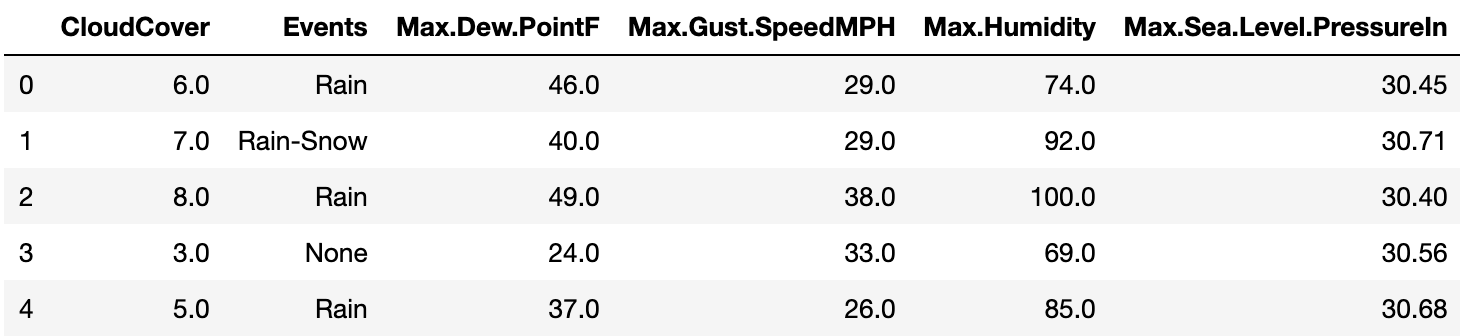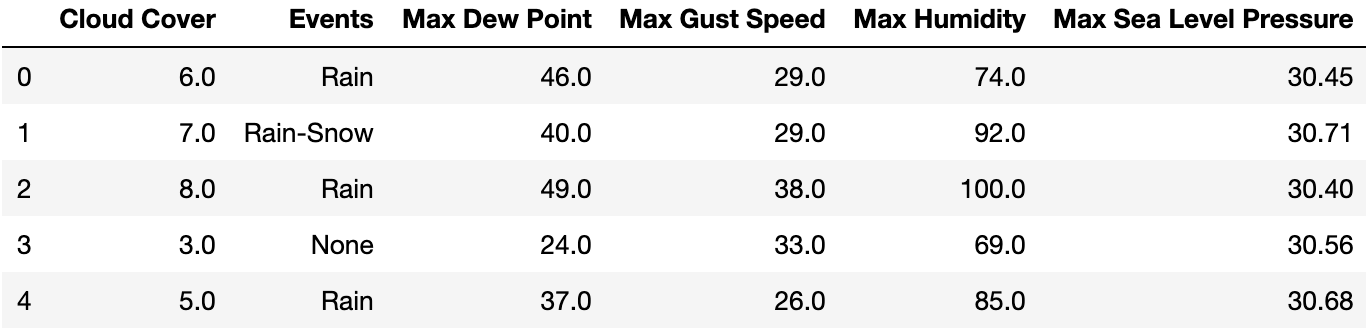Related Tags

data
cleaning
python
pandas

# Basic data cleaning using pandasEducative Answers Team

Prior to performing any sort of data analysis, data scientists spend a lot of time cleaning the dataset to get it in the refined form it needs to be for various data science techniques to be performed on it. It is an important part of a data scientist’s job to handle messy data including missing values, inconsistent formatting, and data types.

The process of data cleaning is roughly covered in the following steps:

• Dropping irrelevant columns.

• Renaming column names to meaningful names.

• Making data values consistent.

• Imputing missing values.

Let’s take a look at how this process can be implemented on pandas data frames in Python.

## 1. Dropping Irrelevant Columns

The drop method of the DataFrame class is used to drop columns from the data frame. See the syntax below:

df.drop([column1, column2, ...], inplace=True, axis=1)

## 2. Renaming column names to meaningful names.

Have a look at the data frame below:It’s a very good approach to rename column names (like these) to ones that can be easily recalled. Have a look at the code below to see how to change the column names of the df data frame.

columns = ["Cloud Cover", "Events", "Max Dew Point", "Max Gust Speed", "Max Humidity", "Max Sea Level Pressure"]
df.columns = columns## 3. Making data values consistent.

Have a look at the column below:

Texture
loam
lOAm
lom
looam

The value in all four rows is supposed to be the same (i.e., “loam”). However, there are different versions of this single value. These data value variations need to be made consistent. The most efficient way to do this is by using regex. Take a look at the example below that replaces all variations of the word with “loam.”

df["Texture"] = df["Texture"].replace(to_replace ="^l.*", value = 'loam', regex = True)


## 4. Imputing missing values

Run the following line of code to see the number of missing values in each column of the dataframe:

df.isnull().sum()


There are various ways to imputate missing values of a column, but replacing null values with the 50th percentile value of the column is the most widely used method. See the code below:

df.loc[df["column"].isnull(),"column"] = df["column"].quantile(0.5)


Other approaches make use of the backfill and forward fill methods for missing value imputation. The mode or the mean value of the column can also used to replace the empty cells.

RELATED TAGS

data
cleaning
python
pandas